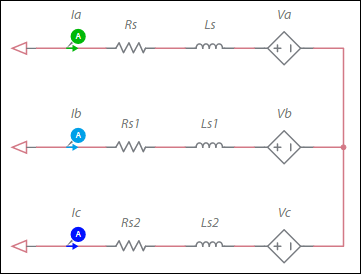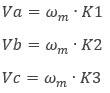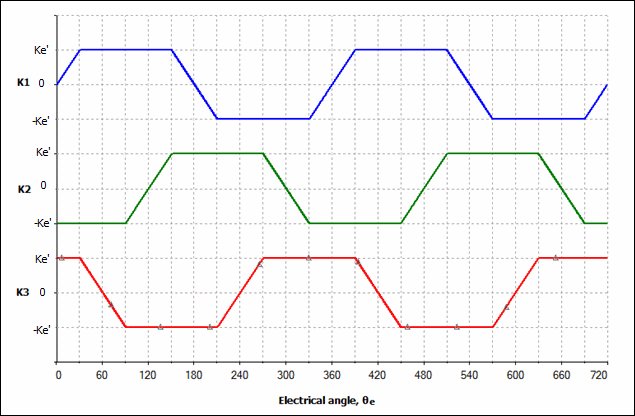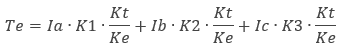# Brushless DC machine model

Model assumptions

• Linear iron magnetization with no saturation
• Constant self and mutual inductance
• Uniform air-gap
• No slot harmonics
• Winding arrangement that produces a perfectly trapezoidal back-EMF waveform (at constant speed)
• No zero phase sequence (system is balanced)

Configuration of the machine electrical systemwhere

ωm = mechanical angular speed
K1, K2, K3 = speed factors (see figure below)

The figure below illustrates speed factor vs. angle waveforms:θe = θm * P

where

θe = electrical angle
θm = mechanical angle
P = number of rotor pole pairs

The generated electromagnetic torque, Te, is:Kt = torque constant
Ke = speed constant

This component contains the following properties in the Model section of the right pane:

Variable Description
Stator inductance Inductance of the stationary portion of the motor.
Stator resistance Resistance of the stationary portion of the motor.
Speed constant The plateau value of the trapezoidal waveform in the diagram above is Ke′=Ke/2
Torque constant Typically equal in value to the speed constant. (Kt in the generated electromagnetic torque equation in Configuration of the Machine Electrical System above.)
Number of pole pairs Number of rotor pole pairs.
Shaft inertia Inertia of the shaft in kg•m<sup>2</sup>. This is J<sub>rotor</sub> on the machine model diagram in <a href="/help/components/machine-modeling/">Machine modeling</a>.
Shaft friction This is F<sub>rotor</sub> on the machine model diagram in <a href="/help/components/machine-modeling/">Machine modeling</a>.
Initial angular speed Rotational measurement of the shaft angle in rad/s at the start of the simulation.
Initial angle Initial shaft angle in radians.